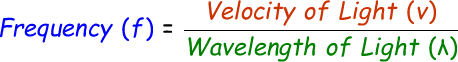# Wavelength to Frequency Converter

The conversion of wavelength to frequency can be done when you know the wavelength of light and the velocity of the light.

We can convert wavelength to frequency using this below formula:where,
f = Frequency of the light [Hz]
v = Velocity of the light [m/s]
λ = Wavelength of the light [nm]

In the below online wavelength to frequency calculator, enter the velocity and wavelength of the light and click calculate button to find the answer.

 Velocity of the Light (v): [m/s] Wavelength of the Light (λ): [nm] Frequency of the Light (f): [Hz]

Latest Calculator Release

Average Acceleration Calculator

Average acceleration is the object's change in speed for a specific given time period. ...

Free Fall Calculator

When an object falls into the ground due to planet's own gravitational force is known a...

Torque Calculator

Torque is nothing but a rotational force. In other words, the amount of force applied t...

Average Force Calculator

Average force can be explained as the amount of force exerted by the body moving at giv...

Angular Displacement Calculator

Angular displacement is the angle at which an object moves on a circular path. It is de...